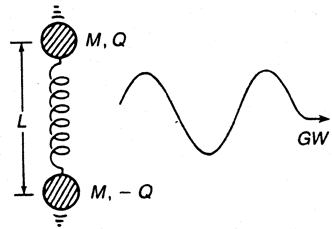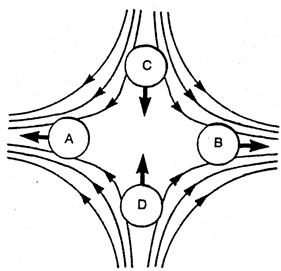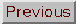Stephen P. Boughn

In the language of Albert Einstein's general theory of relativity, gravitational radiation or gravitational waves (GWs) are ``ripples in the geometry of space and time.'' A less abstruse way to describe gravitational radiation is by drawing an analogy to the electromagnetic spectrum (light, infrared, radio, microwave, x-ray, etc.). Just as these represent forms of the free radiation or waves associated with electricity and magnetism, so GWs represent the radiation associated with the force of gravity. Einstein actually predicted their existence in 1916, the same year his paper on general relativity theory was published. He even calculated the radiation emitted from a binary star system (the strongest source known at the time) and concluded that the radiation was so weak that it had ``a negligible practical effect.'' For the next half century gravitational radiation remained a theoretical curiosity that was of no practical astrophysical significance. In the last two decades astrophysicists have discovered several new potential sources and have come to believe that it not only may be possible to detect gravitational waves directly but also that their emission may even be the dominant process in the evolution of some astrophysical objects.

### GENERATION AND DETECTION

Electromagnetism and gravity are the only two fundamental, longrange forces in nature. Just as accelerated electric charges generate electromagnetic radiation, so do accelerated ``gravitational charges,'' that is, masses, generate gravitational radiation. Simply by analogy with electromagnetism, it is not surprising that gravitational waves are predicted by general relativity and every other viable theory of gravity. Furthermore, if gravity is to obey the laws of Einstein's special theory of relativity, then gravitational radiation must travel at the speed of light.

Because all electric charges have mass, one might expect gravitational radiation to be as abundant as electromagnetic radiation; however, this is not the case. Consider the system indicated in Fig. 1 that depicts two particles of the same mass M and opposite electric charge ±Q oscillating at opposite ends of a spring of length L. The ratio of the power PG emitted in gravitational radiation to the power PEM emitted in electromagnetic radiation by this system is

PG / PEM = (GM2 / Q2)(L /)2

whereis the wavelength of the radiation. If the particles are electrons (which are responsible for most of the electromagnetic radiation we observe), the first factor alone is 10-43, which illustrates the incredible weakness of gravity. The second term in the preceding equation is proportional to the square of the ratio of the speed of the masses to the speed of light and is always less than 1. From this example it is clear that large, rapidly moving masses are the best sources of GWs.Figure 1. Oscillating masses (electric charges) are a source of gravitational (electromagnetic) waves. This same system can also absorb energy from an incident gravitational (electromagnetic) wave.

Electromagnetic radiation is detected by a wide variety of instruments, all of which operate on the same principle: electromagnetic waves exert force on electric charges. Likewise, gravitational radiation can be detected by the force it exerts on masses. If a GW is incident on the spring system of Fig. 1 the masses will be driven into oscillation. The amplitude of the oscillations, however, is very small, again because of the weakness of gravity. In fact, the ratio of the energy absorbed from a gravitational wave to the energy absorbed from an electromagnetic wave of equal strength is given by exactly the same ratio as in the equation.Figure 2. Lines of force of a plane, polarized gravitational wave in the plane perpendicular to the direction of propagation of the wave. The resultant accelerations of four test masses, A, B, C, and D are indicated by bold arrows.

As is characteristic of electromagnetic waves, GWs have two possible polarizations and exert force on matter only in directions perpendicular to the direction of propagation of the wave. The energy in the wave decreases inversely with the square of the distance from the source. For a polarized GW the lines of force in the plane perpendicular to the direction of propagation are illustrated in Fig. 2. The resultant accelerations of four test masses, A, B, C, and D, are indicated by arrows. One-half cycle later the directions of the lines of force and accelerations are reversed. A representation of the other possible polarization is obtained by rotating the figure by 45°. The primitive gravitational wave detector of Fig. 1 is obtained by simply connecting masses A and B (or C and D) by a spring.

### SOURCES

Considering the inherent weakness of gravity, laboratory sources of gravitational radiation are nonexistent. For example, a 1-ton steel bar spun so rapidly that it is on the verge of being ripped apart by centrifugal force radiates less than 10-30 W. (This problem was considered by Einstein in 1918.) By contrast, existing detectors are only sensitive enough to detect such a source (at a distance of one wavelength) if it emits more than 106 W. Current hopes for directly detecting GWs are pinned on astrophysical sources in which massive bodies undergo tremendous accelerations. Short term binary star systems emit strongly (1025-1029 W) at frequencies from 10-4-10-3 mHz, but there are currently no detectors capable of detecting such sources even nearby. Even though not directly detectable, the energy loss from the short term binary pulsar PSR 1913+16 due to gravitational radiation has been measured by precise timing observations of the pulsar orbit. These measurements agree with the predictions of general relativity to within 1%, a result which is an important confirmation of the existence of GWs. A much stronger source is gravitational collapse to a black hole during which a large fraction of the mass of an entire star may be accelerated to velocities approaching the speed of light. It is expected that as much as 1049 W of GWs will be emitted from such a source in the form of a pulse of duration 0.001 s. It has also been conjectured that massive black holes (105-109 M) are located in the nuclei of many galaxies and quasars. During their formation these objects could emit as much as 1054 J of energy in the form of GWs at frequencies between 10-1 and 10-5 Hz. Several more exotic sources of low frequency gravitational radiation have been suggested, for example, quantum gravity fluctuations in the early universe, a quantum chromodynamic phase transition from free quarks into nucleons and oscillating cosmic strings. The period of GWs from these sources range from days to years and are out of the range of laboratory detectors.

### DETECTORS

The first attempts to detect gravitational waves were made in the 1960s by Joseph Weber who acoustically suspended a massive (1400-kg) aluminum cylinder and monitored the level of excitation of its lowest vibrational mode. Resonant at 1660 Hz, this detector was designed to be sensitive to the burst of gravitational radiation predicted to accompany the formation of a solar mass black hole. His 1969 claim of detection of GWs was not confirmed and it is now the consensus of opinion within the scientific community that GWs have not yet been directly observed. Several cryogenically cooled cylinders are now in operation with a sensitivity adequate to detect the GWs emitted from gravitational collapse occurring anywhere in the Milky Way galaxy. None has been detected. Laser interferometers that detect the relative displacement of two mirrors (separated by hundreds of meters) of a Michelson interferometer are potentially more sensitive detectors that respond to a wider range of frequencies. It is hoped that several of these instruments will be in operation in the 1990s with a sensitivity sufficient to detect gravitational collapse in galaxies outside our own. At lower frequencies (10-3 Hz), radar ranging of spacecraft and the monitoring of solid Earth vibrations have been employed but these methods are not yet sensitive enough to detect such events as the formation of massive black holes in distant galaxies. The effect of GWs on the arrival times of pulsars has been used to place upper limits on a background of GWs with periods of a few years. These limits already have provided important constraints on some models of cosmic strings.

### GRAVITATIONAL WAVE ASTRONOMY

The observations of the binary pulsar PSR 1913+16 have established the existence of gravitational radiation, but as an astrophysical source of GWs this system is the least interesting object imaginable, that is, two point particles in orbit around one another. The main motivation of researchers in the field is not simply to observe GWs directly and thereby confirm their existence, but rather to be able to use them to probe deeply into the regions of strong gravitational fields and dense matter that may block other forms of radiation. Only when GWs are detected from one or more of the spectacular astrophysical phenomena mentioned above will gravitational wave astronomy be considered a legitimate branch of astrophysics.